# Simple Data Statistics (Temperature)

Arduino collects and calculates basic statistics on the acquired analog (temperature) data: average, standard deviation, min and max.

BeginnerProtip5,798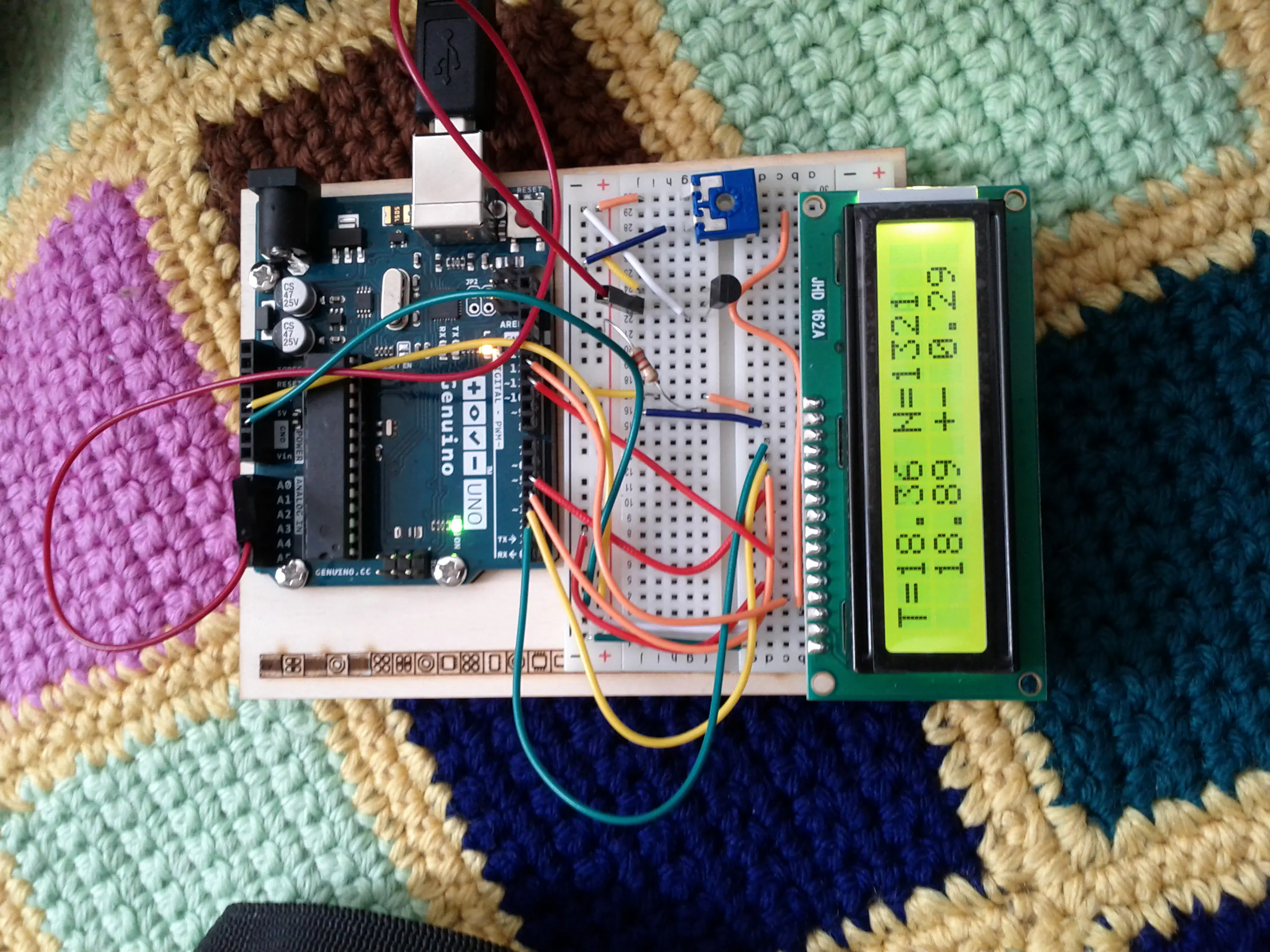## Things used in this project

### Hardware componentsArduino UNO
×1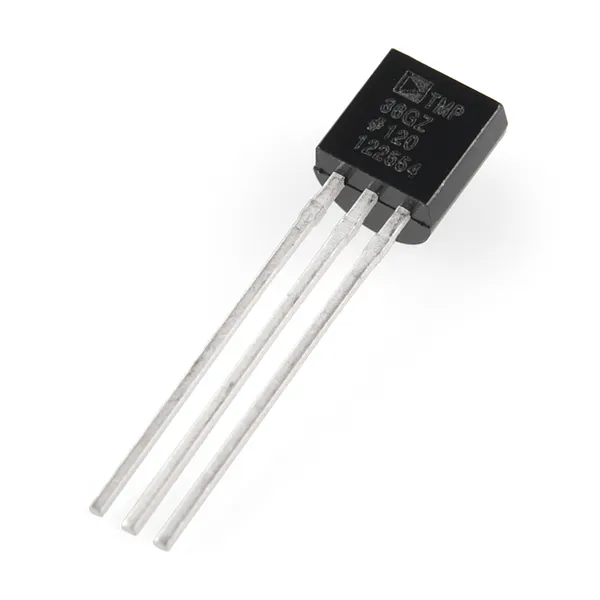Temperature Sensor
×1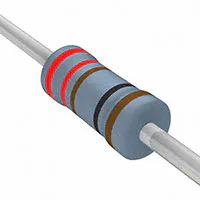Resistor 221 ohm
×1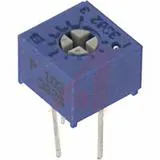Single Turn Potentiometer- 10k ohms
×1Jumper wires (generic)
×1×1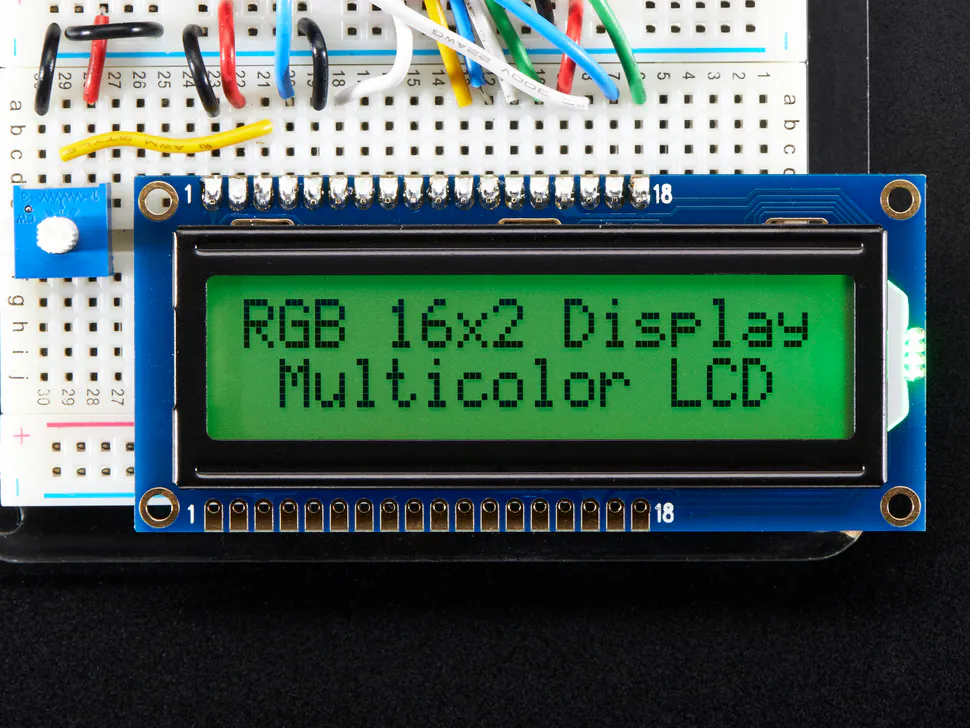Adafruit RGB Backlight LCD - 16x2
×1

## Schematics

### Schematic

There is a Genuino board, a LCD 16x2 and a LMP36 temperature sensors and a lot of wires!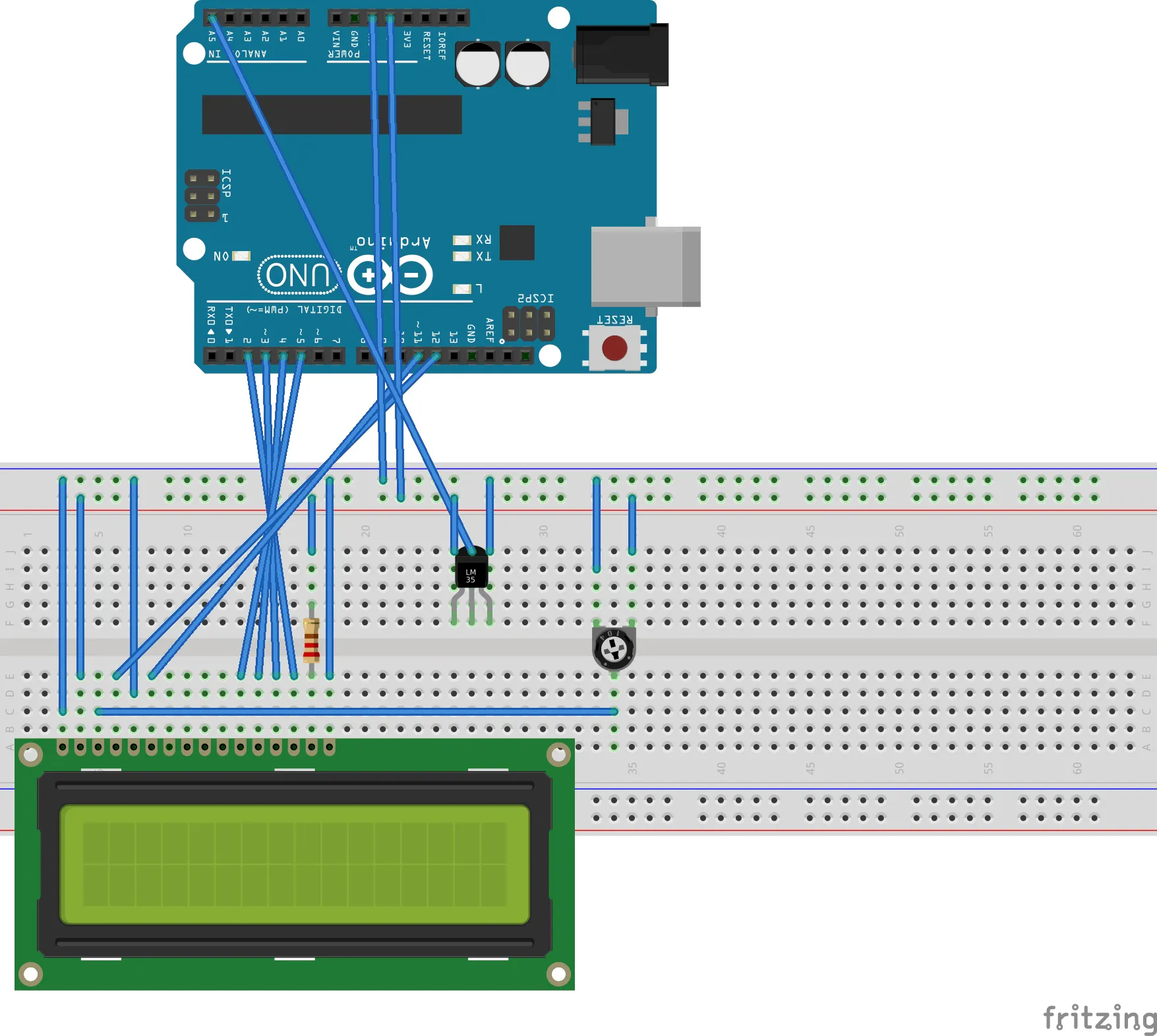## Code

### AvgStd.ino

C/C++
main file.
```#include "AvgStd.h"
#include <LiquidCrystal.h>

LiquidCrystal lcd(12,11,5,4,3,2);
const int sensorPin = A0;
AvgStd mySamples;

void setup(){
mySamples = AvgStd();
mySamples.setRejectionSigma(7.0);
Serial.begin(9600);
while (!Serial) {
; // wait for serial port to connect. Needed for native USB port only
}

// send an intro:
Serial.println("\n\nAvgStd:");
Serial.println();
lcd.begin(16, 2);
// Print a message to the LCD.
lcd.print("hello, world!");
}

void loop(){

// print string
//
lcdPrint(T);

//if reset button pressed
// mySamples.reset();
delay(1000);
}

// convert to V (ADC 1024 values, 5V max)
// float vr = ((float)v / 1024.0) * 5.0;
// T = ( v [V] - 0.5 V) * 100 [C]
return 100 * ((((float)v / 1024.0) * 5.0) - 0.5);
}

void serialPrint(float T){
Serial.print("T = " + String(T, 2));
Serial.println(" N = " + String(mySamples.getN()));
Serial.print("avg: " + String(mySamples.getMean(),2));
Serial.println(" +- " + String(mySamples.getStd(),2));
Serial.print("min: " + String(mySamples.getMin(),2));
Serial.println(" max: " + String(mySamples.getMax(),2));

}

void lcdPrint(float T){
lcd.clear();
// print first row with avg/std
lcd.setCursor(0,0);
String a = "T=" + String(mySamples.getMean(),2);
lcd.print(a);
lcd.setCursor(8,0);
a = "+- " + String(mySamples.getStd(),2);
lcd.print(a);
lcd.setCursor(15,0);
lcd.print( mySamples.getN()%2 == 0? "* " : "  " );
// second row alternates min/max with current reading
lcd.setCursor(0,1);
if ((mySamples.getN()/10)%2 == 0){
//display avg+std
lcd.setCursor(0,1);

a = "  " + String(T,2) + " N=" + String(mySamples.getN());
lcd.print(a);

} else {
// display min/max
a = "m " + String(mySamples.getMin(),2);
lcd.print(a);
lcd.setCursor(9,1);
a = "M " + String(mySamples.getMax(),2);
lcd.print(a);
}

}
```

### AvgStd.h

C/C++
Header for the simple iterative statistic package
```/**
* Library to calculate the average and standard deviation of a
* reading iteratively: without storing the whole data set.
*
* Stores:
* double average
* double variance
* double min
* double max
**/

/*

Permission is hereby granted, free of charge, to any person obtaining a copy of this software and associated documentation files (the "Software"), to deal in the Software without restriction, including without limitation the rights to use, copy, modify, merge, publish, distribute, sublicense, and/or sell copies of the Software, and to permit persons to whom the Software is furnished to do so, subject to the following conditions:

The above copyright notice and this permission notice shall be included in all copies or substantial portions of the Software.

THE SOFTWARE IS PROVIDED "AS IS", WITHOUT WARRANTY OF ANY KIND, EXPRESS OR IMPLIED, INCLUDING BUT NOT LIMITED TO THE WARRANTIES OF MERCHANTABILITY, FITNESS FOR A PARTICULAR PURPOSE AND NONINFRINGEMENT. IN NO EVENT SHALL THE AUTHORS OR COPYRIGHT HOLDERS BE LIABLE FOR ANY CLAIM, DAMAGES OR OTHER LIABILITY, WHETHER IN AN ACTION OF CONTRACT, TORT OR OTHERWISE, ARISING FROM, OUT OF OR IN CONNECTION WITH THE SOFTWARE OR THE USE OR OTHER DEALINGS IN THE SOFTWARE.
*/

#ifndef AVGSTD_H
#define AVGSTD_H

#include "Arduino.h"

class AvgStd {
public:
float getMean();
float getStd();
float getMin();
float getMax();
float getVariance();
unsigned int getN();
void reset();
void setRejectionSigma(float);
int getTrend();
void setTrendInterval(int);
void setSamplingInterval(int);
AvgStd();
private:
float min, max, var, avg, r_sigma;
unsigned int N;

};

#endif
```

### AvgStd.cpp

C/C++
implementation of simple statistics
```/**
* Library to calculate the average and standard deviation of a
* reading iteratively: without storing the whole data set.
*
* Stores:
* double average
* double variance
* double min
* double max
**/

/*

Permission is hereby granted, free of charge, to any person obtaining a copy of this software and associated documentation files (the "Software"), to deal in the Software without restriction, including without limitation the rights to use, copy, modify, merge, publish, distribute, sublicense, and/or sell copies of the Software, and to permit persons to whom the Software is furnished to do so, subject to the following conditions:

The above copyright notice and this permission notice shall be included in all copies or substantial portions of the Software.

THE SOFTWARE IS PROVIDED "AS IS", WITHOUT WARRANTY OF ANY KIND, EXPRESS OR IMPLIED, INCLUDING BUT NOT LIMITED TO THE WARRANTIES OF MERCHANTABILITY, FITNESS FOR A PARTICULAR PURPOSE AND NONINFRINGEMENT. IN NO EVENT SHALL THE AUTHORS OR COPYRIGHT HOLDERS BE LIABLE FOR ANY CLAIM, DAMAGES OR OTHER LIABILITY, WHETHER IN AN ACTION OF CONTRACT, TORT OR OTHERWISE, ARISING FROM, OUT OF OR IN CONNECTION WITH THE SOFTWARE OR THE USE OR OTHER DEALINGS IN THE SOFTWARE.
*/

#include "AvgStd.h"
#include <math.h>

AvgStd::AvgStd(){
AvgStd::reset();
}

if (N < 10)
else {
if (r_sigma == -1)
else {
if (abs(avg - val) <= (r_sigma * sqrt(var)) )
}
}

}

if (N == 0) {
avg = val;
min = val;
max = val;
} else if (N == 1) {
//set min/max
max = val > max? val : max;
min = val < min? val : min;

float thisavg = (avg + val)/2;
// initial value is in avg
var = (avg - thisavg)*(avg-thisavg) + (val - thisavg) * (val-thisavg);
avg = thisavg;
} else {
// set min/max
max = val > max? val : max;
min = val < min? val : min;

float M = (float)N;

var = var * ((M-1)/M) + ((val - avg)*(val - avg)/(M+1)) ;

avg = avg * (M/(M+1)) + val / (M+1);
}
N++;
}

void AvgStd::reset(){
N = 0;
avg = 0;
var = 0;
min = 0;
max = 0;
r_sigma = -1;
}

float AvgStd::getMean(){return avg;}
float AvgStd::getStd() {
float ret = -1;
if (N>1)
ret = sqrt(var);
return ret;
}
float AvgStd::getVariance() {return var;}
unsigned int AvgStd::getN(){return N;}
float AvgStd::getMin() {return min;}
float AvgStd::getMax() {return max;}

void AvgStd::setRejectionSigma(float sigmas){
r_sigma = sigmas;
};

```